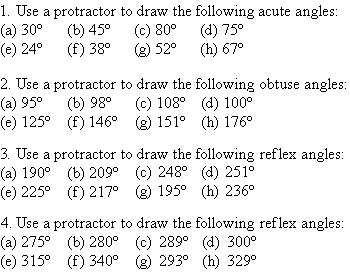Year 7 Interactive Maths - Second Edition

## Drawing Angles less than 180° with a Protractor

To draw an angle with a protractor, proceed as follows:
• Draw a straight line (i.e. an arm of the angle).
• Place a dot at one end of the arm.  This dot represents the vertex of the angle.
• Place the centre of the protractor at the vertex dot and the baseline of the protractor along the arm of the angle.
• Find the required angle on the scale and then mark a small dot at the edge of the protractor.
• Join the small dot to the vertex with a ruler to form the second arm of the angle.
• Label the angle with capital letters.

#### Example 1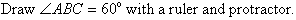##### Solution: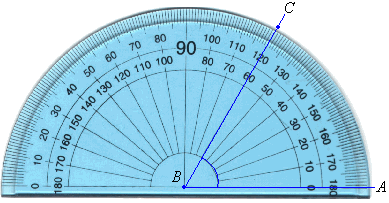• Draw a straight line AB.
• Place a dot at B.  This dot represents the vertex of the angle.
• Place the centre of the protractor at B and the baseline of the protractor along the arm BA.
• Find 60º on the scale and mark a small dot at the edge of the protractor.
• Join the vertex B to the small dot with a ruler to form the second arm, BC, of the angle.
• Mark the angle with a small arc as shown below.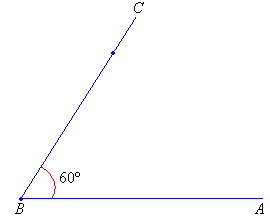## Drawing Reflex Angles

To draw a reflex angle (i.e. angle greater than 180º and less than 360º), proceed as follows:

• Subtract the reflex angle from 360º.  Then draw the resulting angle as described earlier.
• The required angle is outside the one that has been drawn.
• Mark the angle with a small arc.
• Label the angle.

#### Example 2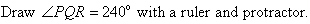##### Solution:• Draw a straight line PQ.
• Place a dot at Q.  This dot represents the vertex of the angle.
• Turn the protractor upside down and place the centre of the protractor at Q and the baseline of the protractor along the arm PQ.
• Use the outer scale to find 120º and mark a small dot at the edge of the protractor.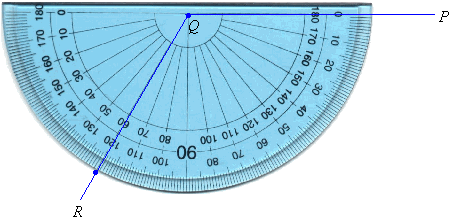• Then remove the protractor and join the vertex, Q, to the small dot with a ruler to form the second arm, QR, of the angle.
• Mark the angle with a small arc as shown below.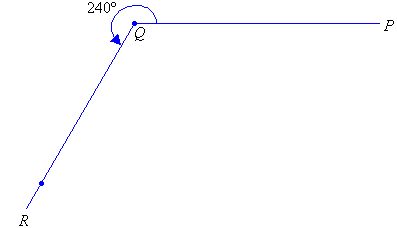### Activity 1